E-mail
New member
New customer
Discover our services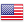Settings
Dynamic quotes
OFF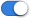ON
 Investment themes The sun, an inexhaustible source of energy
Investment themes
For several years now, the terms "sustainable development" or "green energy" have been gaining popularity. Many companies in the energy sector (in the broadest sense) are working to produce electricity or fuels in a sustainable way to preserve our natural resources.

Today, the protection of our wonderful ecosystem is a major issue that must be taken seriously. However, "greenwashing" is a term often used to denounce the actions of large polluting companies that seek to improve their image through green investments. In this list, we have gathered the main actors who produce components, install or operate solar power plants. Some of these companies are known to be in a transition phase and are still far from being solar pure-players.

Photovoltaics is surely one of the technologies that has attracted the most capital in recent years. It uses cells made of doped semiconductors, which allow the movement of electrons under the effect of light. But photovoltaics is not the only way to harness solar energy. For example, it is possible to heat fluids to very high temperatures using concentrating mirrors. The fluid in question then circulates through turbines and allows us to produce our much needed electricity.

Beyond that, every day we use the energy coming directly from the sun, whether it is to light or heat our home and our work space. Every 50 minutes, the sun provides enough energy to meet the annual consumption of the world population. This is why technologies must be developed both in the production of energy and in its conservation (insulation for example).

This list identifies companies whose main activity is to install or operate solar power plants. The companies selected stand out for their fundamentals and the potential for appreciation of their stock price. The list is regularly updated and only the most qualitative stocks are selected based on their growth, financial health, profitability, valuation (and many other fundamental criteria) among the hundreds of stocks listed worldwide.To access our selection of stocks, regularly updated with new opportunities, discover these tailor-made subscriptions featuring our exclusive tools >> Discover
 Sector Capi. (M\$) 1st Jan %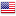░░░░ ░░░ ░░░░░░ ░░░░░ ░░░░░░░░░░░░ ░░░░░ ░░░░░░░ ░ ░░░░░░░░░ ░ ░░░ ░.░░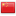░░░░░░░ ░░░░░░ ░░░░░ ░░░░░░░ ░░░░░ ░░░░░░░░░░░░ ░░░░░░░░ ░ ░░░░░░░░ ░ ░░░ ░.░░░░░░░░░░ ░░░░░ ░░░░░░░ ░░░░░░░░ ░░░░ ░░░░ ░░░░░░░░ ░░ ░░░ -░░.░░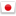░░░░ ░░░░░░░░ ░░░░░░░░░░░ ░░░░░ ░░░░░░░░ ░░░░░░░░░ ░ ░░░ -░░.░░░░░░░░ ░░░░░░ ░░ ░░░░░░░░ ░░░░░░░ ░ ░░░ ░░.░░░░░░░░░ ░░░░░░░░░ ░░░░░░░░░░░░░ ░░░░ ░░░░ ░░░░░ ░░░░░░░░░░░░░░ ░░ ░░░ -░.░░░░░░░░░ ░░░░ ░░░░░ ░░░░░░░░ ░░░░░░░░░ ░ ░░░ -░░.░░░░░░░░░░ ░░░░░ ░░░░ ░░░░░░░░░░░░ ░░░░░ ░░░░░░░ ░ ░░░░░░░░░ ░ ░░░ -░░.░░░░░░░░░ ░░░░ ░░░░ ░░░░░░░░░░░░ ░░░░░ ░░░░░░░ ░ ░░░░░░░░░ ░░ ░░░ -░.░░░░░░░ ░░░░░ ░░░░░░ ░░░░░░░░░░ ░░░░ ░░░░ ░░░░░ ░░░░░░░░░░░░░░ ░░ ░░░ -░.░░░░░░░░░ ░░░░░ ░░░░░░ ░░░░ ░░░░ ░░░░░ ░░░░░░░░░ ░░░░░░ ░░░░░░░░░ ░ ░░░░░░░░ ░░ ░░░ -░░.░░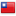░░░░░░░░░░░░░ ░░░░░░░ ░░░░░░░░ ░░░░ ░░░░░ ░░░░░░░░░░░░░░ ░ ░░░ -░.░░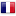░░░░░░░░░░░░░ ░░ ░░░░░░░░░░ ░░░ ░ ░░░ ░░░ ░░░ ░░.░░░░░░░░░ ░░░░░░░ ░░░░ ░░░░░░░░░░░░ ░░░░░ ░░░░░░░ ░ ░░░░░░░░░ ░░ ░░░ -░░.░░░░░░░░░░░ ░░░░░░░░░░░░░ ░░░░ ░░░░░░░░░░░░ ░░░░░ ░░░░░░░ ░ ░░░░░░░░░ ░░ ░░░ -░░.░░░░░░░░░░░░ ░░░░░ ░░░░░░░░░░░░░ ░░░░░░░░ ░ ░░░░░░░░░ ░░░ -░░.░░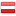░░░░░░░ ░░ ░░░░░ ░░░░░░░░ ░░░░░░░░░ ░░ ░░░ -░.░░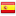░░░░░░░░ ░░░░ ░░░░░ ░░░░░░░░░░░░ ░ ░░░░░░░░░░░ ░ ░░░ -░.░░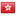░░░░ ░░░░░ ░░░░░ ░░░░ ░░░░ ░░░░░░░░░░░░ ░░░░░ ░░░░░░░ ░ ░░░░░░░░░ ░░ ░░░ -░░.░░░░░░░░░ ░░░░░░░ ░ ░░░░░ ░░░░░░░░░ ░░░░ ░░░░░░░░░ ░░░░ ░ ░░░ -░.░░░░░░░ ░░░░░ ░░░ ░░░░░░ ░░░░░░░ ░░░░░░░ ░░░░░░░░░░░░ ░░░░░ ░░░░░░░ ░ ░░░░░░░░░ ░░░ -░.░░░░░░░░░░ ░░░ ░░░ ░░░░░░ ░░░░░░░░░░ ░░░░░░░░░░░ ░░░░░░░░░░░░░ ░░░░░░░░░ ░░░░░░░░░░░░░ ░ ░░░ -░░.░░░░░░░ ░░░░░ ░░░░░░░░ ░░░░░░░ ░░░░░░░░░░░░ ░░░░░ ░░░░░░░ ░ ░░░░░░░░░ ░░ ░░░ -░.░░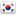░░░░░░ ░░░░░░░░░ ░░░░░░░░░░░ ░░░░░░░░░░░░ ░░░░░ ░░░░░░░ ░ ░░░░░░░░░ ░ ░░░ ░.░░░░░░░░░░░░░ ░░ ░░░░░░ ░░░░░ ░░░░░░░░░ ░░░░░░░░░ ░░ ░░░ -░░.░░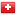░░░░░ ░░░░░░ ░░░░░░░░░░ ░░ ░░░░░░░░░░░░░ ░░░░░░░░░ ░░░░░░░░░░░░░ ░ ░░░ -░░.░░░░░░░░░░ ░░ ░░░░░░░░░░░ ░░░░░░░░ ░░░░░░░░░ ░ ░░░ -░░.░░░░░░░░░░ ░░░░░░░░░░░ ░░░░ ░░░░░░░░░ ░░░░░░ ░░░░░░░░ ░░░ -░.░░░░░░░ ░░░░░░ ░░░░░░░░ ░░░░░░░ ░░░░░░░░░ ░░░░ ░ ░░░ -░.░░░░░░░░░░ ░░░░░░░░ ░░░░░░░░░░░ ░░░░░ ░░░░░░░░░░ ░░░░░░░░░ ░ ░░░░░░░░░ ░ ░░░ -░.░░░░░░ ░░ ░░░░░ ░░░░░░░░░ ░░░░░░░░░ ░░ ░░░ -░.░░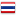░░░░░░ ░░░░░░░░ ░░░░░░ ░░░░░░░ ░░░░░░░ ░░░░░░░░░░░ ░░░░░░░░ ░░░░░░░░░ ░░ ░░░ -░.░░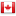░░░░░░░░░ ░░░░░ ░ ░░░░░░░░░ ░░░░░ ░░░░░ ░░░░░░░░░ ░░░░░░░░░ ░ ░░░ -░.░░░░░░░ ░░░░░ ░░░░░░ ░░░░░░░ ░░░░░ ░░░░░░░░ ░░░░░░░░░░ ░░░ -░░.░░░░░░░░░░ ░░░ ░░░░░░░░ ░░░░░░░░░░░░ ░░░ ░.░░░░░░░░░░░ ░░░░ ░░░░░░░░░ ░░░░░░ ░░░░░░░░ ░ ░░░ -░░.░░░░░░░░░░ ░░░░ ░░░░░ ░░░ ░ ░░░ ░░░░░░░░░░░░░░ ░░░░░░░░ ░░ ░░░ ░.░░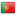░░░ ░░░░░░░░░░░ ░░░░ ░░░░░░░░░ ░░░░ ░░ ░░░ -░░.░░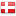░░░░░░ ░░░ ░░░░░ ░░░░░░░░ ░░░░░░░░░ ░░ ░░░ -░░.░░░░░░░ ░░░░░ ░░░░░░░░░ ░░░░░░░░░ ░░ ░░░ ░.░░░░░░░ ░░░░░ ░░░░ ░░░ ░░░░░░░░░░░░ ░░░░░ ░░░░░░░ ░ ░░░░░░░░░ ░░ ░░░ -░░.░░░░░░░░░░ ░░░░░ ░░░░░░░░░░░ ░░░░░░ ░░░░░░░ ░░░░░░░ ░░░░░░░░░ ░░░░ ░░░ ░.░░░░░░░░░░░░ ░░░░░░░ ░░░░ ░░░░ ░░░░░░░░░░░░ ░░░░░ ░░░░░░░ ░ ░░░░░░░░░ ░ ░░░ -░.░░░░░░░░░░ ░░░░░░░░░░░ ░░░░░░░░░░░░ ░░░░░ ░░░░░░░ ░ ░░░░░░░░░ ░ ░░░ -░.░░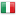░░░░░ ░░░░░░░░░░ ░░░░░░ ░░░░░░░░░ ░░░░ ░ ░░░ ░.░░░░░░░ ░░░░░░ ░░░░ ░░░░░ ░░░░░░░░░ ░░░░░░ ░░░░░░░░░ ░ ░░░░░░░░ ░ ░░░ -░.░░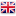░░░ ░░░ ░░░░░ ░░░░░░░░ ░░░░░░░░░ ░░ ░░░ -░.░░░░░░░░░░░░░░ ░░░░░░░ ░░░░ ░░░░░ ░░░░░░░░ ░░░░░░░░░ ░░ ░░░ -░.░░░░░ ░░░░░░ ░░░░░ ░░░░░░░░░░░ ░░░░░ ░░░░░░░░░ ░ ░░░ -░.░░░░░░░░ ░░░░░ ░░░░░░░░░ ░░░░ ░░░░░░░░░ ░░░░░░ ░░░░░░░░ ░░░ -░.░░░░░░ ░░░░░░░░░░░ ░░░░░░░░░░ ░░░░░░░░ ░░░ -░.░░░░░░ ░░░░░░ ░░░░░░░░░░░░ ░░░░░ ░░░░░░░ ░ ░░░░░░░░░ ░░░ -░░.░░░░░░░░ ░░░░░░░░░░░░ ░░░░░░ ░░░░ ░░░░░ ░░░░░░░░░ ░░░░░░ ░░░░░░░░░ ░ ░░░░░░░░ ░ ░░░ -░░.░░░░ ░░░░░ ░░░░░░░░░░ ░░░░ ░░░░ ░░░░░░░░░░░░ ░░░░░ ░░░░░░░ ░ ░░░░░░░░░ ░░ ░░░ -░.░░░░░░░░ ░░░░░ ░░░░░░░░░░░░░ ░░░░ ░░░░░░░░░░░░ ░░░░░ ░░░░░░░ ░ ░░░░░░░░░ ░░░ -░░.░░░░░░░░ ░░░░░░░░░ ░░░░░░░░░░░ ░░░░░░░░░░░░░░ ░░░░░░░░ ░░░░ ░░░░░ ░░░░░░░░░░ ░░░░░ ░ ░░░ -░░.░░░░░░░░░░░░░░░░ ░░░ ░░░░░░ ░░░░░░░░░░░░░ ░░░░ ░░░░░ ░░░░░░░░░░░░ ░ ░░░░░░░░░░░ ░░░ -░.░░░░░░░░░░ ░░░░░░░ ░░░░ ░░░░░ ░░░░░░░░░░░ ░░░░░ ░░░░░░░░░ ░ ░░░ -░.░░░░░ ░ ░░░░░░░░ ░░ ░░░░░░░░░ ░░░░ ░░░░░ ░░░░░░░░ ░░░░░░░░░ ░░ ░░░ -░.░░░░░ ░░░░░ ░░░░░░░░░░ ░░ ░░░░░ ░░░░░░░░░░░░░░ ░ ░░░ -░░.░░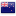░░░░░░░░ ░░░░░░ ░░░░░░░ ░░░░░░░░░░░░░ ░ ░░░░░ ░░░░░░░░░ ░ ░░░ -░.░░░░░░░░░░░ ░░░░░░░ ░░░░ ░░░░ ░░░░░ ░░░░░░░░░ ░░░░░░ ░░░░░░░░░ ░ ░░░░░░░░ ░░░ -░.░░░░░░░░░ ░░░░░ ░░░░░░░ ░░░░ ░░░░ ░░░░░░░░░░░░ ░░░░░ ░░░░░░░ ░ ░░░░░░░░░ ░ ░░░ -░░.░░░░░░░ ░░░░░░ ░░░░░░░░░░░ ░░░░░ ░░░░░ ░░░░░░░░░░ ░░░░░░░░░ ░ ░░░ -░░.░░░░░░░ ░░░░░ ░░░░░░░░░ ░░░░░░ ░░░░░░░░░ ░ ░░░░░░░░ ░ ░░░ -░.░░░░░░░ ░░░░░░░░░░░░░ ░░░░ ░░░░░ ░░░░░░░░░ ░░░░░░ ░░░░░░░░░ ░ ░░░░░░░░ ░ ░░░ -░░.░░░░░░░░░ ░░░░░░ ░░░░░ ░░░░░░░░ ░░░░░░░░░ ░░░ ░░░ -░░.░░░░░░░░░░░ ░░░░ ░░░░░ ░░░░░░░░ ░░░░░░░░░ ░░ ░░░ -░.░░░░░░░░░░░ ░░░░░░░░░░ ░░░░ ░░░░ ░░░░░░░░ ░░░░░░░░░ ░ ░░░ -░.░░
 Heatmap % Price Change by Sector 1 day 5 days 1 week 1 month 3 months 6 months 1 year 3 years 5 years 10 years 1st jan. #heatmap_liste_thema .highcharts-tooltip>span { background-image: linear-gradient(#FFFFFF,#EEEEEE); border-radius: 5px; padding: 8px; z-index: 10; } .highcharts-tooltip>span a { color: #004EFF !important; } Sector allocation Ratings chart - Surperformance Ratings ESG Refinitiv